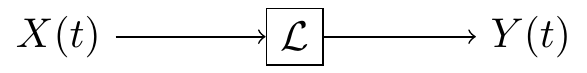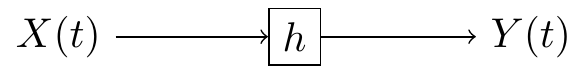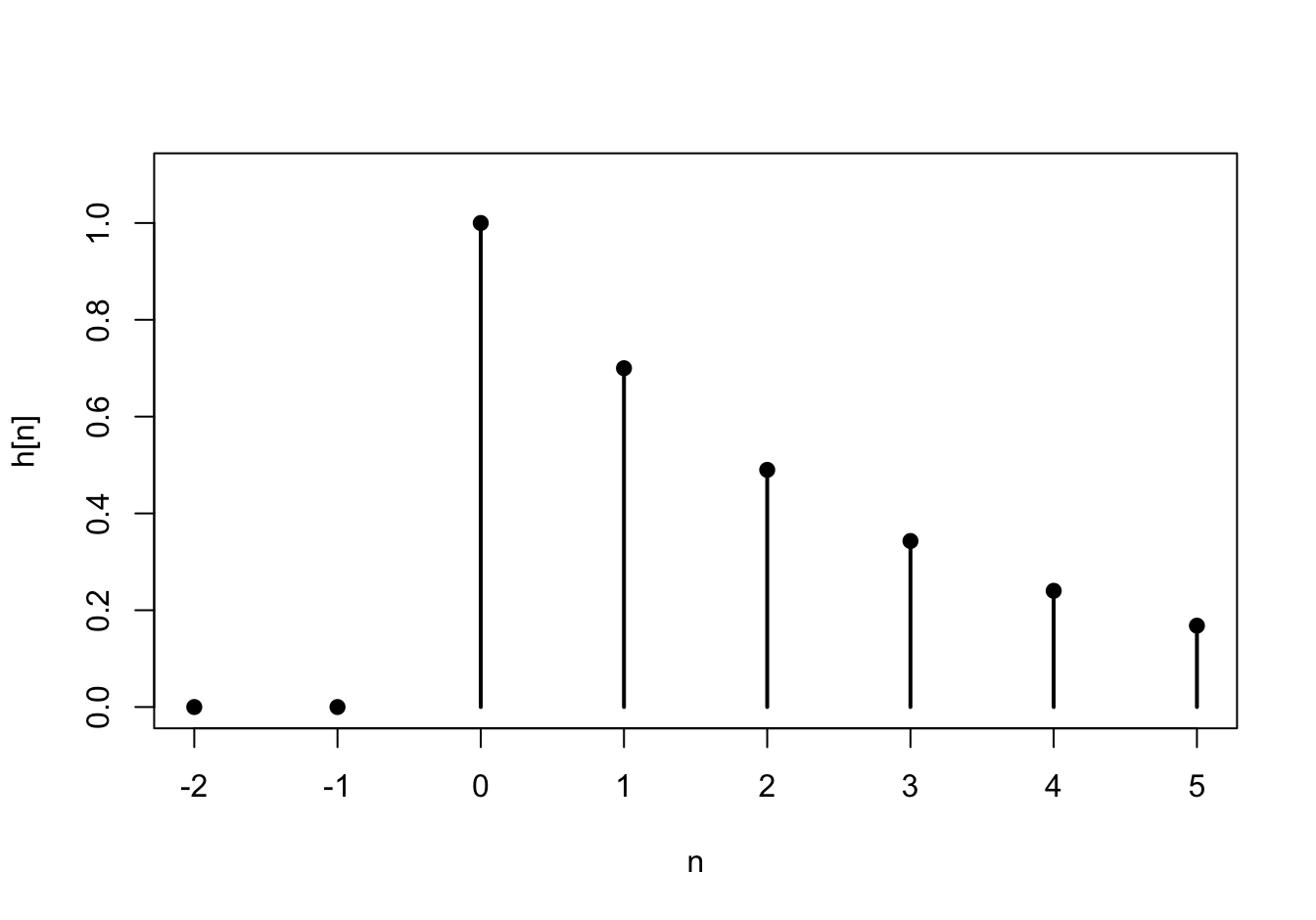# Lesson 57 LTI Filters in the Time Domain

## Motivation

What happens when we pass a stationary random signal $$\{ X(t) \}$$ into a filter $$\mathcal{L}$$? This lesson studies the properties of the output signal $$\{ Y(t) \}$$, which is also a random process.Figure 57.1: Filtering a Signal

## Review

We begin this lesson by recalling a few basic facts about linear time-invariant filters. This material can be found in any signals and systems textbook.

Definition 57.1 (Linear Time-Invariant Filter) A filter $$\mathcal{L}$$ takes an input signal $$x(t)$$ and produces an output signal $$y(t)$$.

In general, a filter can do anything to a signal. We will restrict our attention to a specific class of filters called linear time-invariant (or LTI, for short) filters. An LTI filter satisfies the following properties:

1. linearity: $$\mathcal{L}[a_1x_1 + a_2x_2] = a_1\mathcal{L}[x_1] + a_2\mathcal{L}[x_2]$$ for any constants $$a_1, a_2$$ and signals $$x_1, x_2$$.
2. time-invariance: $$\mathcal{L}[x(t - \tau)](t) = \mathcal{L}[x](t - \tau)$$ for any shift $$\tau$$. In other words, if the input signal $$x(t)$$ is delayed by $$\tau$$ seconds, then the output signal $$y(t)$$ is also delayed by $$\tau$$ seconds, without any other modifications.

Theorem 57.1 (Impulse Response) Let $$h(t)$$ denote the impulse response of an LTI filter $$\mathcal{L}$$. That is, $$h \overset{\text{def}}{=} \mathcal{L}[\delta]$$ is the output signal when the input signal is an impulse $$\delta(t)$$.

Then, $$\mathcal{L}$$ acts by convolving $$h$$ with the input signal $$x$$. That is, $y(t) = (h * x)(t).$

Proof. We will prove this result for discrete-time signals, although the proof for continuous-time signals is similar.

First, write $$x = x * \delta$$. Any signal convolved with a delta function is itself. This is called the sifting property of the delta function. To see why this is true, write out the definition of convolution: $x[n] = \sum_{m=-\infty}^\infty x[m] \delta[n-m].$ All terms in this sum will be zero, except when $$m=n$$, when the term is $$x[n] \delta = x[n]$$.

From here, we just apply linearity and time-invariance. \begin{align*} y[n] = \mathcal{L}\big[x[n]\big][n] &= \mathcal{L}\Big[\sum_{m=-\infty}^\infty x[m] \delta[n - m]\Big][n] & \text{(sifting property)} \\ &= \sum_{m=-\infty}^\infty x[m] \mathcal{L}\big[\delta[n-m]\big][n] & \text{(linearity)} \\ &= \sum_{m=-\infty}^\infty x[m] h[n-m] & \text{(time-invariance)}. \end{align*} This is just the definition of convolution: $$h * x$$.

Because the impulse response uniquely describes any LTI filter, it is common to redraw Figure 57.1, using $$h$$ to symbolize the filter.Figure 57.2: Linear Time-Invariant Filter, represented by its impulse response $$h$$

Example 57.1 (Infinite Impulse Response (IIR) Filter) Consider the discrete-time filter defined by $y[n] = a_1 y[n-1] + x[n].$ Find the impulse response of this filter.

Solution. The impulse response $$h[n]$$ is the output of the filter when the input is the unit impulse $$\delta[n]$$. That is, if $$x[n] = \delta[n]$$, then $$y[n] = h[n]$$.

Therefore, we have the relation $h[n] = a_1 h[n-1] + \delta[n]$ for all $$n$$.

First, we observe that $$h[n] = 0$$ for all $$n < 0$$. For $$n \geq 0$$, we can calculate $$h[n]$$ recursively: \begin{align*} h &= a_1 h[-1] + \delta = a_1 \cdot 0 + 1 = 1 \\ h &= a_1 h + \delta = a_1 \cdot 1 + 0 = a_1 \\ h &= a_1 h + \delta = a_1 \cdot a_1 + 0 = a_1^2 \\ h &= a_1 h + \delta = a_1 \cdot a_1^2 + 0 = a_1^3 \\ &\vdots \end{align*} At this point, the pattern is hopefully clear. We can write the impulse response as $h[n] = \begin{cases} a_1^n & n \geq 0 \\ 0 & n < 0 \end{cases} = a_1^n u[n],$ where $$u[n]$$ is the unit step function, defined to be 1 when $$n \geq 0$$ and 0 otherwise. This impulse response is graphed below for $$a_1 = 0.7$$.## Theory

We are now ready to apply linear filters to stationary random processes.

Theorem 57.2 (Filtering Stationary Processes) Let $$\{ X(t) \}$$ be a stationary random process and $$h(t)$$ be the impulse response of a linear time-invariant filter. If $$\{ X(t) \}$$ is passed into a linear time-invariant filter with impulse response $$h(t)$$, then the output process $$\{ Y(t) \}$$ is also stationary, with

• mean function $$\mu_Y = \mu_X \cdot \int_{-\infty}^\infty h(t)\,dt$$, and
• autocovariance function $$C_Y(\tau) = (h(-t) * h * C_X)(\tau)$$.

For discrete-time signals, we have:

• mean function $$\mu_Y = \mu_X \cdot \sum_{k=-\infty}^\infty h[k]$$, and
• autocovariance function $$C_Y[k] = (h[-n] * h * C_X)[k]$$.

Proof. We will prove the theorem for discrete-time signal, but the proof for continuous-time signals is similar.

The mean function is \begin{align*} \mu_Y[n] = E[Y[n]] &= E\left[\sum_{k=-\infty}^\infty h[k] X[n-k] \right] \\ &= \sum_{k=-\infty}^\infty h[k] \underbrace{E[X[n-k]]}_{\mu_X} \\ &= \mu_X \sum_{k=-\infty}^\infty h[k]. \end{align*}

The autocovariance function is \begin{align*} C_Y[m, n] = \text{Cov}[Y[m], Y[n]] &= \text{Cov}\left[\sum_{k=-\infty}^\infty h[k] X[m-k], \sum_{\ell=-\infty}^\infty h[\ell] X[n - \ell] \right] \\ &= \sum_{k=-\infty}^\infty \sum_{\ell=-\infty}^\infty h[k] h[\ell] \text{Cov}[X[m-k], X[n-\ell]] \\ &= \sum_{\ell=-\infty}^\infty h[\ell] \sum_{k=-\infty}^\infty h[k] C_X[(m-n) + \ell - k] \\ &= \sum_{\ell=-\infty}^\infty h[\ell] (h * C_X)[(m-n) + \ell] \\ &= \sum_{\ell=-\infty}^\infty h[-\ell] (h * C_X)[(m-n) - \ell] \\ &= (h[-n] * h * C_X)[m-n] \end{align*}

Since the mean function is constant and the autocovariance function only depends on $$m-n$$, $$\{ Y[n]\}$$ is also stationary.

Example 57.2 (Autoregressive Process) Let $$\{ Z[n] \}$$ be white noise, consisting of i.i.d. random variables with mean $$0$$ and variance $$\sigma^2$$. Then, the process $X[n] = a_1 X[n-1] + Z[n]$ is called an autoregressive process (of order 1). We will derive the autocovariance function of $$\{ X[n] \}$$ using Theorem 57.2.

Notice that $$\{ X[n] \}$$ is the output signal when white noise $$\{ Z[n] \}$$ is passed into the LTI filter from Example 57.1. That is, $$\{ X[n] \}$$ is the output when a stationary process is passed into a filter with impulse response $h[n] = (0.8)^n u[n].$ Therefore, we know that $$\{ X[n] \}$$ is also stationary.

Its mean function is $$\displaystyle \mu_X = \mu_Z \sum_k h[k] = 0$$, since we assumed that $$\{ Z[n] \}$$ was mean 0.

Its autocovariance function, as a function of the difference $$k=m-n$$, is $C_X = h[-n] * h * C_Z.$ We calculated the autocovariance function of white noise in Example 52.3: $C_Z[k] = \sigma^2 \delta[k].$ Now, applying the sifting property of the delta function, we see that $C_X = h[-n] * h * C_Z = \sigma^2 (h[-n] * h).$ So we just need to convolve the impulse response $$h[n]$$ with a time-reversed version $$h[-n]$$. \begin{align*} (h[-n] * h)[k] &= \sum_{m=-\infty}^\infty h[m] h[-(k-m)] & \text{(definition of convolution)} \\ &= \sum_{m=-\infty}^\infty a_1^{m} u[m] a_1^{m-k} u[m-k] & \text{(definition of h)} \\ &= \sum_{m=-\infty}^\infty a_1^{2m-k} u[m] u[m-k] & \text{(combining terms)} \end{align*} Notice that the unit step function $$u$$ will be zero unless both $$m \geq 0$$ and $$m \geq k$$. If $$k$$ is non-negative, then the second condition implies the first. So $$u[m] u[m-k]$$ has the effect of changing the limits of the sum: \begin{align*} &= \sum_{m=k}^\infty a_1^{2m-k}; k \geq 0 \\ &= \frac{a_1^k}{1 - a_1^2}; k \geq 0 & \text{(infinite geometric series)} \end{align*}

Putting it all together, when $$k$$ is non-negative, the autocovariance function of $$\{ X[n] \}$$ is $C_X[k] = \sigma^2 \frac{a_1^k}{1 - a_1^2}; k \geq 0.$ But covariance is symmetric, so $$C_X[k] = C_X[-k]$$. Therefore, we can say in general that $C_X[k] = \sigma^2 \frac{a_1^{|k|}}{1 - a_1^2}.$

This example illustrates the power of Theorem 57.2. Although the derivation of the autocovariance function was quite involved, this derivation is still more straightforward than any of the alternatives.

## Essential Practice

1. Find the power spectral density of the autoregressive process, in terms of $$a_1$$ and $$\sigma$$. (Hint: Use the mean function and autocovariance function that we derived in Example 57.2.) Graph the power spectral density for $$a_1 = 0.8$$ and $$\sigma = 2$$.

2. Consider the moving average process $$\{ X[n] \}$$ of Example 48.2, defined by $X[n] = 0.5 Z[n] + 0.5 Z[n-1],$ where $$\{ Z[n] \}$$ is a sequence of i.i.d. standard normal random variables.

Express $$\{ X[n] \}$$ as white noise passed through an LTI filter. What is the impulse response of this filter? Then, use Theorem 57.2 to calculate the mean and autocovariance functions of $$\{ X[n] \}$$. Check that your answers agree with the ones you obtained in Lessons 50 and 52.